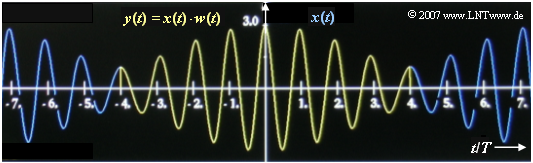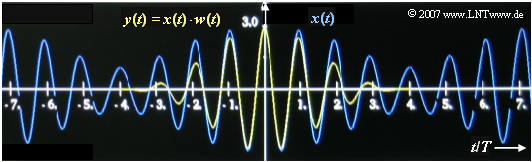# Exercise 5.4: Comparison of Rectangular and Hanning Window

Let the time course of a periodic signal be given in principle:

$$x(t) = A_1 \cdot \cos (2 \pi \cdot f_1 \cdot t) + A_2 \cdot \cos (2 \pi \cdot f_2 \cdot t) \hspace{0.05cm}.$$

Unknown and thus to be estimated are its parameters  $A_1$,  $f_1$,  $A_2$  and  $f_2$.

After weighting the signal with the window function  $w(t)$ , the product  $y(t) = x(t) \cdot w(t)$  is subjected to a  Discrete Fourier Transform  (DFT) with the parameters  $N = 512$  and  $T_{\rm P}$.  The time  $T_{\rm P}$  of the signal section to be analyzed can be set by the user as desired.

Two functions are available for windowing, each of which is zero for  $|t| > T_{\rm P}/2$:

• The  rectangular window:
$${w} (\nu) = \left\{ \begin{array}{c} 1 \\ 0 \\ \end{array} \right.\quad \begin{array}{*{10}c} {\rm{for}} \\ \\ \end{array}\begin{array}{*{20}c} -N/2 \le \nu < N/2 \hspace{0.05cm}, \\ {\rm else} \hspace{0.05cm}, \\ \end{array}$$
$$W(f) ={1}/{f_{\rm A}}\cdot {\rm si}(\pi \cdot {f}/{f_{\rm A}})\hspace{0.05cm},$$
• the  Hanning window:
$${w} (\nu) = \left\{ \begin{array}{c} 0.5 + 0.5 \cdot \cos (2 \pi \cdot {\nu}/{N}) \\ 0 \\ \end{array} \right.\quad \begin{array}{*{10}c} {\rm{for}} \\ \\ \end{array}\begin{array}{*{20}c} -N/2 \le \nu < N/2 \hspace{0.05cm}, \\ {\rm else} \hspace{0.05cm}, \\ \end{array}$$
$$W(f) ={0.5}/{f_{\rm A}}\cdot {\rm si}(\pi \cdot \frac{f}{f_{\rm A}})+ {0.25}/{f_{\rm A}}\cdot {\rm si}(\pi \cdot \frac{f-f_{\rm A}}{f_{\rm A}})+ {0.5}/{f_{\rm A}}\cdot {\rm si}(\pi \cdot \frac{f+f_{\rm A}}{f_{\rm A}})\hspace{0.05cm}.$$

Here, $W(f)$  is the Fourier transform of the continuous-time window function  $w(t)$, while  $w(ν)$  indicates the discrete-time weighting function.

In the task, reference is made to various spectral functions  $Y(f)$  for example to

$$Y_{\rm A}(f) = 1\, {\rm V}\cdot {\rm \delta} (f \pm 1\,\,{\rm kHz})+ 0.5\,\, {\rm V}\cdot {\rm \delta} (f \pm 1.125\,\,{\rm kHz}) \hspace{0.05cm}.$$

In the graph, two further spectral functions  $Y_{\rm B}(f)$  and  $Y_{\rm C}(f)$  are shown, which result when a  $1 \ \text{kHz}$  signal is analyzed by DFT and the DFT parameter  $T_{\rm P} = 8.5 \ \text{ms}$  is chosen unfavourably.

• For one of the images the rectangular window is used, for the other the Hanning window.
• It is not indicated which graph belongs to which window.

Hints:

• This task belongs to the chapter  Spectrum Analysis.
• Note that the frequency resolution  $f_{\rm A}$  is equal to the reciprocal of the adjustable parameter  $T_{\rm P}$.
• Unfortunately, the indices of  $f_{\rm A}$  and  $Y_{\rm A}(f)$ collide.  It is obvious that they are not related.  Just to be on the safe side, we point this out.

### Questions

1

Which of the following statements are true with certainty when the DFT displays the output spectrum  $Y_{\rm A}(f)$ ?

 The rectangular window was used for weighting. The Hanning window was used for weighting. The DFT parameter  $T_{\rm P} = 4\ \text{ms}$  was used. The DFT spectrum  $Y_{\rm A}(f)$  is identical to the actual spectrum  $X(f)$.

2

Using the Hanning window and  $T_{\rm P} = 8 \ \text{ms}$, what is  $Y(f)$  when the input spectrum  $X(f) = Y_{\rm A}(f)$  is applied?
Give the weights of the Dirac lines at  $f_1= 1\ \text{kHz}$   ⇒   $G(f_1)$  and at   $f_2 = 1.125\ \text{kHz}$  ⇒   $G(f_2)$.

 $G(f_1 = 1.000 \ \text{kHz})\ = \$  $\text{V}$ $G(f_2 = 1.125 \ \text{kHz})\ = \$  $\text{V}$

3

We consider the  $1\ \text{kHz}$ cosine signal  $x(t)$.  Which spectrum -  $Y_{\rm B}(f)$  or  $Y_{\rm C}(f)$  – results with the rectangular or the Hanning window, respectively, if the DFT parameter  $T_{\rm P} = 8.5 \ \text{ms}$  is chosen unfavourably?

 $Y_{\rm B}(f)$  results with rectangular windowing. $Y_{\rm B}(f)$  results with the Hanning window.

### Solution

#### Solution

(1)  Solutions 1 and 4 are correct:

• Using the Hanning window, three Dirac functions should be recognisable even if  $x(t)$  contains only one frequency   ⇒   the rectangular window was used.
• With  $T_{\rm P} = 4 \ \text{ms}$ , the frequency resolution is  $f_{\rm A}= 1/T_{\rm P} = 0.25 \ \text{kHz}$.  Thus the frequency  $f_2$  does not lie in the given grid and  $Y(f)$  would be composed of very many Dirac lines.  This means:   the third statement is wrong.Output signal  $y(t)$  with the rectangular window
• As can be seen from the graph,  $x(t)$  has the period duration  $T_{\rm 0} = 8 \ \text{ms}$.
• If one chooses the DFT parameter equal to  $T_{\rm P} = 4 \ \text{ms}$  (or an integer multiple thereof), the periodic continuation  ${\rm P}\{ x(t)\}$  in the interval  $|t| \leq T_{\rm P}/2$  coincides with  $x(t)$ , so that the weighting function  $w(t)$  has no disturbing effect.  So:
• The DFT spectrum  $Y(f)$  thus agrees with the actual spectrum.

(2)  Because of  $T_{\rm 0} = 8 \ \text{ms}$ , the Hanning spectrum  $W(f)$

• consists of three Dirac functions at positive frequencies
• and three axisymmetrical Diracs at negative frequencies

are composed. For the positive frequencies, the spectral function is:Output signal  $y(t)$  with the Hanning window
$$W(f) =0.5\cdot {\rm \delta}(f) + 0.25\cdot {\rm \delta}(f-f_{\rm A})+ 0.25\cdot {\rm \delta}(f+f_{\rm A})\hspace{0.05cm}.$$

The output spectrum results from the convolution between  $X(f)$  and  $W(f)$.  At positive frequencies, there are now four Diracs with the following weights:

\begin{align*} G(f = 0.875\,{\rm kHz}) & = 1\, {\rm V}\cdot 0.25 = 0.250\, {\rm V}, \\ G(f = f_1 = 1.000\,{\rm kHz}) & = 1\, {\rm V}\cdot 0.5 + 0.5\, {\rm V}\cdot 0.25 \hspace{0.15 cm}\underline{ = 0.625\, {\rm V}}, \\ G(f = f_2 = 1.125\,{\rm kHz}) & = 1\, {\rm V}\cdot 0.25 + 0.5\, {\rm V}\cdot 0.5 \hspace{0.15 cm}\underline{= 0.500\, {\rm V}}, \\ G(f = 1.250\,{\rm kHz}) & = 0.5\, {\rm V}\cdot 0.25 = 0.125\, {\rm V} \hspace{0.05cm}.\end{align*}

The graph shows the attenuation of the edges by the weighting function  $w(t)$  of the Hanning window.

(3)  Solution 2 is correct:

• The rectangular window delivers a very strongly distorted result if the window width  $T_{\rm P}$  (as here) is not adapted to the cosine frequency.
• In this case, the Hanning window is more suitable.  Then the measured spectrum  $Y_{\rm B}(f)$ results.
• From the spectrum  $Y_{\rm C}(f)$  the searched $1\ \rm kHz$ line is more difficult to detect.  The spectrum  $Y_{\rm C}(f)$  results after rectangular windowing.